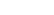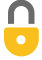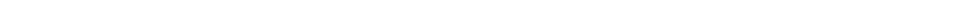Problem 29
Q

# Estimate how long it would take one person to mow a football field using an ordinary home lawn mower (Fig. 1–15). (State your assumption, such as the mower moves with a 1-km/h speed, and has a 0.5-m width.)Figure 1-15.A
11hrIn order to watch this solution you need to have a subscription.VIDEO TRANSCRIPT

This is Giancoli Answers with Mr. Dychko. A football field is 360 feet long if you include the end zones and 160 meters wide or sorry 160 feet wide I should say. So we need to express everything in meters because we are gonna assume that the molar width is half a meter wide and you can walk with a speed of 1 kilometer per hour. So the width in meters is 160 feet times 1 meter for every 3.28 feet and that gives 48.78 meters is the total width of the football field. So the number of lengths you have to do here with this bush mower— each of these red lines represents a length— so you go across from 1 swathe, 2 swathe, 3 swathe and so on. The number of times you have to go along this football field will be 48.78 meters—total width—times 1 length needed for every half a meter so you only cover half a meter in width for each length and that means you are gonna have to walk the field 97.58 times. And then the total distance you will end up walking then is the number of times you do a length— 97.58 times— multiplied by the number of meters for each length well, it's 360 feet but we have to convert that into meters by multiplying 360 feet by 1 meter for every 3.28 feet and that's the number of meters per length and the lengths cancel here giving us a total distance traveled of 10707 meters. And if you wanna figure out the time then it's gonna be the distance divided by the speed and the speed is 1 kilometer per hour or 1 times 10 to the 3 meters per hour and this 10707.9 meters divided by 10 to the 3 meters per hour is gonna work out to 11 hours to mow the field.